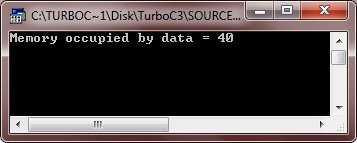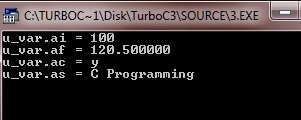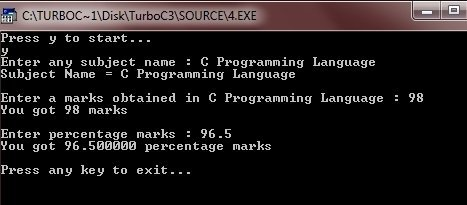# C Unions

As you know that a union is a derived data type. A union is used in a memory location that is shared by two or more different types of variables. A union provides a way of interpreting the same bit pattern in two or more different ways.

## Declare a Union in C

Declaring a union is just similar to declaring a structure. But in declaring a union, use union keyword instead of struct. Here is the general form to define a union in C:

```union tag
{
type member_name;
type member_name;
type member_name;
.
.
.
}union_variables;```

Here, union is a keyword, must be used in declaring a union, tag is an identifier, is simply the name of the union. And then type is any valid data type and member_name is the name of the member. And in last union_variables is an identifier, is the name of variable, used in accessing the union members. Here is an example:

```union u_data
{
int num;
float fl;
char ch;
char str;
}data;```

As you can see from the above declaration, a variable of u_data type can store an integer, a floating-point number, a character, or a string of characters. In other words, a single variable i.e., the same memory location can be used to store the multiple types of data. You can also use any built-in or user-defined data types inside a union.

Note - The memory occupied by a union will be big enough to hold the largest member of the union.

For instance, from the above union declaration, u_data type will occupy 40 bytes of memory space because this is the maximum space which can be occupied by the character string named str.

## Access Union Members in C

Use the member access operator (.) to access any member of a union. Here is the general form to access any member of a union:

`union_variable.member_name;`

Here is an example, showing how to access the members of the union u_data which is declared above.

```data.num;
data.fl;
data.ch;
data.str;```

## Access and Initialize Values to Union Members in C

Here is the general form to access and initialize values to any union members in C:

`union_variable.member_name = value;`

Here is an example, showing how to access and initialize values to the members of the union named u_data which is declared above.

```data.num = 100;
data.fl = 120.50;
data.ch = 'y';
strcpy(data.str, "C Programming");```

Now let's take some examples for complete understanding on unions.

## C Unions Example

Here is an example program illustrating unions in C language. This is s very basic union program. Just concentrate on this.

```/* C Unions */

#include<stdio.h>
#include<string.h>
#include<conio.h>

union u_data
{
int num;
float fl;
char ch;
char str;
}data;

void main()
{
clrscr();

printf("Memory occupied by data = %d\n", sizeof(data));

getch();
}```

On compile and executing the above code, it will produce the following result:You can also declare union variable later. Let's take a look at this C program.

```/* C Unions */

#include<stdio.h>
#include<string.h>
#include<conio.h>

union u
{
int n;
float f;
char c;
char s;
};

void main()
{
union u u_max_size;
clrscr();

printf("Memory occupied = %d\n", sizeof(u_max_size));

getch();
}```

Here is the sample output of the above C program:Here is another example illustrating how to access and initialize values to union members one by one.

```#include<stdio.h>
#include<string.h>
#include<conio.h>

union u
{
int ai;
float af;
char ac;
char as;
};

void main( )
{
union u u_var;
clrscr();

u_var.ai = 100;
printf("u_var.ai = %d\n", u_var.ai);

u_var.af = 120.5;
printf("u_var.af = %f\n", u_var.af);

u_var.ac = 'y';
printf("u_var.ac = %c\n", u_var.ac);

strcpy(u_var.as, "C Programming");
printf("u_var.as = %s\n", u_var.as);

getch();
}```

Below is the sample output of this C program:Now, here is another example demonstrating unions in C.

```#include<stdio.h>
#include<string.h>
#include<conio.h>

union u
{
int num;
float flt;
char ch;
char str;
};

void main()
{
union u u_var;
clrscr();

printf("Press y to start...\n");
scanf("%c", &u_var.ch);
fflush(stdin);
if(u_var.ch=='y' || u_var.ch=='Y')
{
printf("Enter any subject name : ");
gets(u_var.str);
printf("Subject Name = %s", u_var.str);
printf("\n\nEnter a marks obtained in %s : ", u_var.str);
scanf("%d", &u_var.num);
printf("You got %d marks", u_var.num);
printf("\n\nEnter percentage marks : ");
scanf("%f", &u_var.flt);
printf("You got %f percentage marks", u_var.flt);
printf("\n\nPress any key to exit...\n");
getch();
exit(1);
}

getch();
}```

Here is sample run of this C program:Tools
Calculator